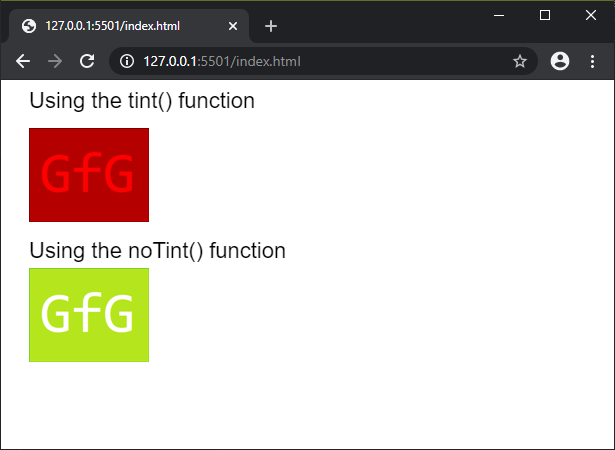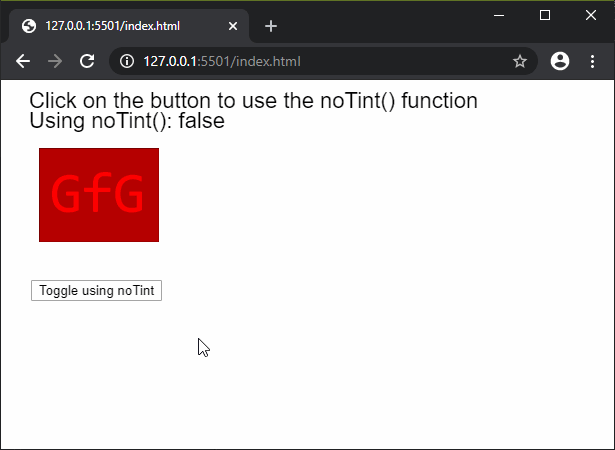# p5.js | noTint() Function

• Last Updated : 02 Mar, 2020

The noTint() function is used to remove the fill value for images that has been previously applied using the tint() function. It will revert the tint of the images and display them with their original hues.

Syntax:

Hey geek! The constant emerging technologies in the world of web development always keeps the excitement for this subject through the roof. But before you tackle the big projects, we suggest you start by learning the basics. Kickstart your web development journey by learning JS concepts with our JavaScript Course. Now at it's lowest price ever!

`noTint()`

Parameters: This function does not accept any parameters.

Below examples illustrate the noTint() function in p5.js:

Example 1:

 `function` `preload() {``  ``img = loadImage(``'sample-image.png'``);``}`` ` `function` `setup() {``  ``createCanvas(600, 300);``  ``textSize(22);``}`` ` `function` `draw() {``  ``clear();``  ``text(``"Using the tint() function"``, 20, 20);``  ``tint(``"red"``);``  ``image(img, 20, 40);`` ` `  ``text(``"Using the noTint() function"``, 20, 170);``  ``noTint()``  ``image(img, 20, 180);``}`

Output:Example 2:

 `function` `preload() {``  ``img = loadImage(``'sample-image.png'``);``  ``disableTint = ``false``;``}`` ` `function` `setup() {``  ``createCanvas(600, 300);``  ``textSize(22);`` ` `  ``// Create a button for toggling the``  ``// noTint() function``  ``removeBtn = createButton(``"Toggle using noTint"``);``  ``removeBtn.position(30, 200)``  ``removeBtn.mousePressed(removeTint);``}`` ` `function` `draw() {``  ``clear();``  ``text(``"Click on the button to use the noTint() function"``, 20, 20);``  ``text(``"Using noTint(): "` `+ disableTint, 20, 40);`` ` `  ``// Check if the boolean value is true``  ``// to use the noTint() function``  ``// in this draw loop``  ``if` `(disableTint) noTint();`` ` `  ``image(img, 30, 60);`` ` `  ``// Using the tint() function here``  ``// would tint the image in the next``  ``// draw loop``  ``tint(``"red"``);``}`` ` `function` `removeTint() {``  ``// Toggle the use of noTint()``  ``disableTint = !disableTint;``}`

Output:Online editor: https://editor.p5js.org/

Reference: https://p5js.org/reference/#/p5/notint

My Personal Notes arrow_drop_up